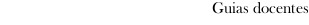Guia docente 2021_22Facultad de Ciencias Económicas y Empresariales
 Degree in EconomicsSubjectsStatistics IIContents
 Topic Sub-topic 1. Introduction 1. Reminder of Statistics I 2. Aims of Statistics II 2. Point estimation 1. Introduction 2. Properties of the estimators 3. Maximum likelihood estimation 3. Interval estimation 1. Introduction 2. Confidence intervals for normal distributions 3. Confidence intervals for proportions 4. Confidence interval for the average of a Poisson distribution 4. Parametric hypothesis testing 1. Introduction 2. Tests for normal distributions 3. Tests for proportions 4. Tests for the average of a Poisson distribution 5. Nonparametric hypothesis testing 1. Introduction 2. Test of randomness 3. Tests of goodness of fit 4. Tests of homogeneity for independent samples 5. Tests of homogeneity for paired samples 6. Test of independence 6. Simple linear regression model 1. Introduction 2. Least square estimators 3. Coefficient of determination (square R) 4. Hypothesis testing and confidence intervals for the parameters of the model 5. Prediction
 Universidade de Vigo            | Rectorado | Campus Universitario | C.P. 36.310 Vigo (Pontevedra) | España | Tlf: +34 986 812 000